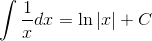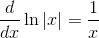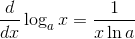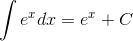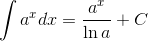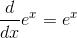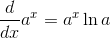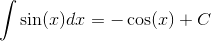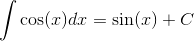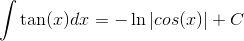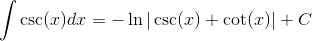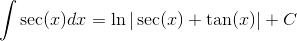### Day 70 - Inverse Functions: Differentiation - 12.02.14

Lesson Objective(s)
• How are derivatives of inverse functions found?

#### In-Class Help Requests

Standard(s)
• APC.9

• Apply formulas to find derivatives.

• Includes:

• derivatives of algebraic, trigonometric, exponential, logarithmic, and inverse trigonometric functions

• derivations of sums, products, quotients, inverses, and composites (chain rule) of elementary functions

• derivatives of implicitly defined functions

• higher order derivatives of algebraic, trigonometric, exponential, and logarithmic, functions

Math
ematical Practice(s)
• #1 - Make sense of problems and persevere in solving them
• #2 - Reason abstractly and quantitatively
• #5 - Use appropriate tools strategically
• #6 - Attend to precision
• #8 - Look for and express regularity in repeated reasoning

Past Checkpoints
• Differentiation of the Natural Logarithmic Function
• Integration with the Natural Logarithmic Function
• Exponential Functions: Differentiation and Integration
• Bases Other Than e: Differentiation and Integration